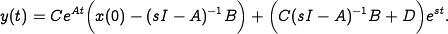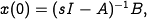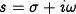# FAQ: In the displayed equation after equation (8.3), how can you set x(0), a constant, to a function of s?

Jump to: navigation, search

(Contributed by Richard Murray, 10 September 2012)

In the derivation of the exponential response of a linear system, equation (8.3) gives the following expression for:To obtain the pure exponential response (the right hand term), the text states that we should choose the initial state asSince we are used to thinking ofas the argument for a transfer function, it may seem confusing to setto a non-constant value.

However, in this derivation we are considering the response of a linear system to a fixed input of the formwhereis a constant of the form. Settingto the value given above thus result in the first term in the exponential response being zero. Note that this value only works for this specific choice of; a different value must be chosen for different exponential inputs.

Also note thatis complex-valued in this expression. This is OK because in practice we will always add together combinations of signals that result in real-valued expressions. See FAQ: Why is the exponential response complex valued? Shouldn't the inputs and outputs of a linear system always be real-valued?